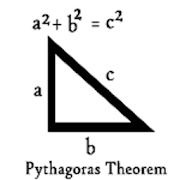## Pythagorean Theorem Calculator helps you practice Grade HS Math - Lumos Educational App StorePrice -fREE

#### DESCRIPTION:

Pythagorean Theorem Calculator is the best calculator to calculate hypotenuse. The Pythagorean Theorem states that aÂ²+bÂ²=cÂ² and can be used to find the length of the hypotenuse of a right triangle. Simply input the length of two sides and the app will calculate the third side according to the Pythagorean Theorem.You can easily find the average with the help of this app .you will get math table upto 100000. Basic Features: Pythagorean Calculator. Average calculator. Math Table. Square & Square Root Calculator Very helpful app for teachers and students. So please download

#### OVERVIEW:

Pythagorean Theorem Calculator is a free educational mobile app By .It helps students in grades HS practice the following standards HSG.SRT.B.4.

This page not only allows students and teachers download Pythagorean Theorem Calculator but also find engaging Sample Questions, Videos, Pins, Worksheets, Books related to the following topics.

1. HSG.SRT.B.4 : Prove theorems about triangles. Theorems include: a line parallel to one side of a triangle divides the other two proportionally, and conversely; the Pythagorean Theorem proved using triangle similarity..

HS

#### STANDARDS:

HSG.SRT.B.4

Software Version: 1

Category:

### RELATED APPS

Rate this App?
0

0 Ratings & 0 Reviews

5
0
0
4
0
0
3
0
0
2
0
0
1
0
0EdSearch WebSearch